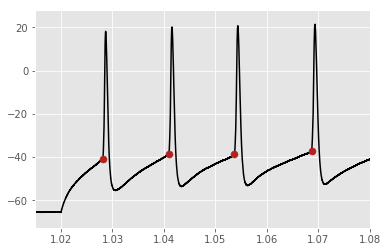## Cell Types Database¶

This notebook demonstrates most of the features of the AllenSDK that help manipulate data in the Cell Types Database. The main entry point will be through the CellTypesCache class.

CellTypesCache is responsible for downloading Cell Types Database data to a standard directory structure on your hard drive. If you use this class, you will not have to keep track of where your data lives, other than a root directory.

In :
from allensdk.core.cell_types_cache import CellTypesCache

# Instantiate the CellTypesCache instance.  The manifest_file argument
# tells it where to store the manifest, which is a JSON file that tracks
# file paths.  If you supply a relative path it will go into your
# current working directory
ctc = CellTypesCache()

# this saves the NWB file to 'cell_types/specimen_464212183/ephys.nwb'
cell_specimen_id = 464212183
data_set = ctc.get_ephys_data(cell_specimen_id)

/local1/anaconda3/envs/py2/lib/python2.7/site-packages/h5py/__init__.py:36: FutureWarning: Conversion of the second argument of issubdtype from float to np.floating is deprecated. In future, it will be treated as np.float64 == np.dtype(float).type.
from ._conv import register_converters as _register_converters


The data_set variable is an NwbDataSet instance, which has some methods we can use to access the injected current stimulus waveform and the voltage response waveform for all experimental sweeps. Let's pull one sweep out and plot it.

In :
%matplotlib inline
import numpy as np
import matplotlib.pyplot as plt

sweep_number = 30
sweep_data = data_set.get_sweep(sweep_number)

index_range = sweep_data["index_range"]
i = sweep_data["stimulus"][0:index_range+1] # in A
v = sweep_data["response"][0:index_range+1] # in V
i *= 1e12 # to pA
v *= 1e3 # to mV

sampling_rate = sweep_data["sampling_rate"] # in Hz
t = np.arange(0, len(v)) * (1.0 / sampling_rate)

plt.style.use('ggplot')
fig, axes = plt.subplots(2, 1, sharex=True)
axes.plot(t, v, color='black')
axes.plot(t, i, color='gray')
axes.set_ylabel("mV")
axes.set_ylabel("pA")
axes.set_xlabel("seconds")
plt.show()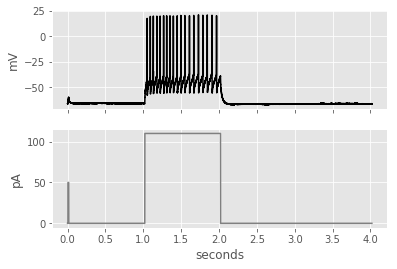Cell records in the Cell Types Database come with a large amount of metadata. We have exposed the most commonly used of these are arguments to CellTypesCache.get_cells.

In :
from allensdk.core.cell_types_cache import CellTypesCache
from allensdk.api.queries.cell_types_api import CellTypesApi
from allensdk.core.cell_types_cache import ReporterStatus as RS

cells = ctc.get_cells()
print("Total cells: %d" % len(cells))

# mouse cells
cells = ctc.get_cells(species=[CellTypesApi.MOUSE])
print("Mouse cells: %d" % len(cells))

# human cells
cells = ctc.get_cells(species=[CellTypesApi.HUMAN])
print("Human cells: %d" % len(cells))

# cells with reconstructions
cells = ctc.get_cells(require_reconstruction = True)
print("Cells with reconstructions: %d" % len(cells))

# all cre positive cells
cells = ctc.get_cells(reporter_status = RS.POSITIVE)
print("Cre-positive cells: %d" % len(cells))

# cre negative cells with reconstructions
cells = ctc.get_cells(require_reconstruction = True,
reporter_status = RS.NEGATIVE)
print("Cre-negative cells with reconstructions: %d" % len(cells))

Total cells: 2333
Mouse cells: 1920
Human cells: 413
Cells with reconstructions: 667
Cre-positive cells: 1815
Cre-negative cells with reconstructions: 38


## Cell Morphology Reconstructions¶

The Cell Types Database also contains 3D reconstructions of neuronal morphologies. The data are presented in the SWC format. We'll download a particular cell's reconstrution here.

The AllenSDK contains a module that makes it easier to work with the SWC files. We'll see how the data is contained in the file by looking at the first node.

In :
import pprint

cell_id = 480114344
morphology = ctc.get_reconstruction(cell_id)

# the compartment list has all of the nodes in the file
pprint.pprint(morphology.compartment_list)

{'children': [1,
1763,
2012,
2089,
2421,
2604,
2821,
3147,
3440,
3491,
3552,
4015],
'id': 0,
'parent': -1,
'tree_id': 0,
'type': 1,
'x': 444.3296,
'y': 503.0168,
'z': 31.92}


Note that the type field refers to the type of neuronal compartment. The values can be 1 for the soma, 2 for the axon, 3 for dendrites, and 4 for apical dendrites (if present).

Morphologies now also come with marker files, which contains points of interest in the reconstruction. The marker file contains locations where dendrites have been truncated due to slicing and when axons were not reconstructed. The name field indicates the type of marker (10 for dendrite truncation, 20 for no reconstruction).

In :
# download and open a marker file
markers = ctc.get_reconstruction_markers(cell_id)
pprint.pprint(markers)

{'name': 10, 'x': 527.5029999999999, 'y': 496.4319, 'z': 12.4555}


We can use this data to draw lines between each node and all its children to get a drawing of the cell. We'll do it looking at it from the front and from the side.

In :
from allensdk.core.swc import Marker
fig, axes = plt.subplots(1, 2, sharey=True, sharex=True)
axes.set_aspect('equal', 'box-forced')
axes.set_aspect('equal', 'box-forced')

# Make a line drawing of x-y and y-z views
for n in morphology.compartment_list:
for c in morphology.children_of(n):
axes.plot([n['x'], c['x']], [n['y'], c['y']], color='black')
axes.plot([n['z'], c['z']], [n['y'], c['y']], color='black')

# cut dendrite markers
dm = [ m for m in markers if m['name'] == Marker.CUT_DENDRITE ]

axes.scatter([m['x'] for m in dm], [m['y'] for m in dm], color='#3333ff')
axes.scatter([m['z'] for m in dm], [m['y'] for m in dm], color='#3333ff')

# no reconstruction markers
nm = [ m for m in markers if m['name'] == Marker.NO_RECONSTRUCTION ]

axes.scatter([m['x'] for m in nm], [m['y'] for m in nm], color='#333333')
axes.scatter([m['z'] for m in nm], [m['y'] for m in nm], color='#333333')

axes.set_ylabel('y')
axes.set_xlabel('x')
axes.set_xlabel('z')
plt.show()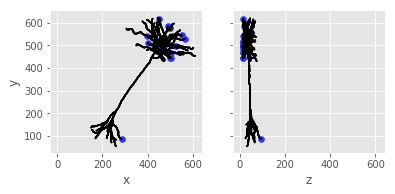## Electrophysiology Features¶

The Cell Types Database contains a set of features that have already been computed, which could serve as good starting points for analysis. We can query the database using the SDK to get these features.

In :
import pandas as pd

ephys_features = ctc.get_ephys_features()
ef_df = pd.DataFrame(ephys_features)

print("Ephys. features available for %d cells" % len(ef_df))

# filter down to a specific cell
specimen_id = 464212183
cell_ephys_features = ef_df[ef_df['specimen_id']== specimen_id]
cell_ephys_features

/local1/git/allensdk/allensdk/api/cache.py:382: FutureWarning: from_csv is deprecated. Please use read_csv(...) instead. Note that some of the default arguments are different, so please refer to the documentation for from_csv when changing your function calls

Ephys. features available for 2333 cells

Out:
adaptation avg_isi electrode_0_pa f_i_curve_slope fast_trough_t_long_square fast_trough_t_ramp fast_trough_t_short_square fast_trough_v_long_square fast_trough_v_ramp fast_trough_v_short_square ... trough_t_ramp trough_t_short_square trough_v_long_square trough_v_ramp trough_v_short_square upstroke_downstroke_ratio_long_square upstroke_downstroke_ratio_ramp upstroke_downstroke_ratio_short_square vm_for_sag vrest
1129 0.01066 50.912222 -73.090617 0.385991 1.243575 3.872615 1.025798 -58.406254 -56.364585 -61.026044 ... 3.87297 1.273295 -58.59375 -56.635419 -65.729168 1.915684 2.045541 1.821178 -90.46875 -65.771713

1 rows × 55 columns

That's how to get all the ephys features for a given specimen - what if we want a particular feature for all cells?

In :
plt.figure()
plt.scatter(ef_df['fast_trough_v_long_square'],
ef_df['upstroke_downstroke_ratio_long_square'], color='#2ca25f')
plt.ylabel("upstroke-downstroke ratio")
plt.xlabel("fast trough depth (mV)")
plt.show()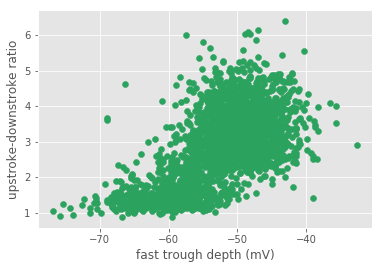Let's use numpy to fit a regression line to these data and plot it.

In :
A = np.vstack([ef_df['fast_trough_v_long_square'],
np.ones_like(ef_df['upstroke_downstroke_ratio_long_square'])]).T

print("First 5 rows of A:")
print(A[:5, :])

m, c = np.linalg.lstsq(A, ef_df['upstroke_downstroke_ratio_long_square'], rcond=None)
print("m", m, "c", c)

plt.figure()
plt.scatter(ef_df['fast_trough_v_long_square'],
ef_df['upstroke_downstroke_ratio_long_square'],
color='#2ca25f')
plt.plot(ef_df['fast_trough_v_long_square'],
m * ef_df['fast_trough_v_long_square'] + c, c='gray')
plt.ylabel("upstroke-downstroke ratio")
plt.xlabel("fast trough depth (mV)")
plt.show()

First 5 rows of A:
[[-56.37500381   1.        ]
[-54.           1.        ]
[-59.5          1.        ]
[-47.53125      1.        ]
[-48.43750381   1.        ]]
('m', 0.10468807187601809, 'c', 8.355078903861308)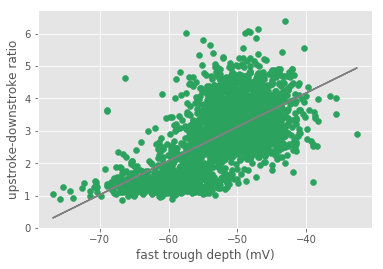It looks like there may be roughly two clusters in the data above. Maybe they relate to whether the cells are presumably excitatory (spiny) cells or inhibitory (aspiny) cells. Let's query the API and split up the two sets to see.

In :
cells = ctc.get_cells()

# we want to add dendrite type as a column to the ephys. features dataframe
# first build an index on cell specimen ID, then create array of dendrite types
cell_index = { c['id']: c for c in cells }
dendrite_types = [ cell_index[cid]['dendrite_type'] for cid in ef_df['specimen_id'] ]

# now add the new column
ef_df['dendrite_type'] = pd.Series(dendrite_types, index=ef_df.index)

fig = plt.figure()

for d_type, color in [ ["spiny", "#d95f02"], ["aspiny", "#7570b3"] ]:
df = ef_df[ef_df['dendrite_type'] == d_type]
plt.scatter(df['fast_trough_v_long_square'],
df['upstroke_downstroke_ratio_long_square'],
color=color, label=d_type)

plt.ylabel("upstroke-downstroke ratio")
plt.xlabel("fast trough depth (mV)")
plt.legend(loc='best')

plt.show()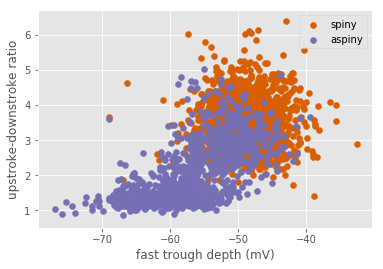## Morphology Features¶

The Cell Types Database contains a set of precomputed morphological features for cells that have reconstructions. You can access morphology features by themselves, or combined with the electrophysiology features.

In :
import pandas as pd

morphology_features = ctc.get_morphology_features()

all_features = ctc.get_all_features(require_reconstruction=True)

# convert to a pandas DataFrame
all_features = pd.DataFrame(all_features)
print("All features available for %d cells" % len(all_features))


All features available for 670 cells

Out:
adaptation average_bifurcation_angle_local average_bifurcation_angle_remote average_contraction average_diameter average_fragmentation average_parent_daughter_ratio avg_isi electrode_0_pa f_i_curve_slope ... trough_t_ramp trough_t_short_square trough_v_long_square trough_v_ramp trough_v_short_square upstroke_downstroke_ratio_long_square upstroke_downstroke_ratio_ramp upstroke_downstroke_ratio_short_square vm_for_sag vrest
0 NaN 72.604110 NaN 0.850561 0.527213 75.133929 0.966709 134.700000 22.697498 8.335459e-02 ... 13.295680 1.13478 -56.593754 -57.739586 -74.143753 3.029695 3.061646 2.969821 -80.468750 -73.553391
1 NaN 68.499396 NaN 0.934072 0.614133 61.172414 0.892380 NaN -24.887498 -3.913630e-19 ... 20.650735 1.16094 -55.406254 -55.242191 -73.500000 2.441895 2.245653 2.231575 -84.406258 -73.056595
2 0.044825 77.679949 NaN 0.895664 0.488865 48.027778 0.928510 91.322222 -46.761876 2.238637e-01 ... 3.862007 1.80651 -50.875000 -50.989586 -76.791669 2.992793 2.817856 2.706951 -96.906250 -75.320374
3 0.002303 73.926537 NaN 0.896227 0.216384 62.282609 0.993927 8.756140 -33.787497 6.366711e-01 ... 16.753540 1.29864 -61.031254 -65.000005 -79.625003 0.987204 1.191762 1.294996 -85.593750 -79.520042
4 0.093492 89.985385 NaN 0.821116 0.289549 54.000000 1.000000 112.245000 3.015000 1.500000e-01 ... 5.483953 1.22590 -51.406254 -59.552087 -81.875008 2.199872 2.220031 2.235442 -95.843758 -81.065971

5 rows × 83 columns

## Computing Electrophysiology Features¶

The AllenSDK contains the code used to compute the electrophysiology features you accessed above. You can run it yourself like this.

In :
from allensdk.ephys.ephys_extractor import EphysSweepFeatureExtractor

sweep_number = 35
sweep_data = data_set.get_sweep(sweep_number)

index_range = sweep_data["index_range"]
i = sweep_data["stimulus"][0:index_range+1] # in A
v = sweep_data["response"][0:index_range+1] # in V
i *= 1e12 # to pA
v *= 1e3 # to mV

sampling_rate = sweep_data["sampling_rate"] # in Hz
t = np.arange(0, len(v)) * (1.0 / sampling_rate)

sweep_ext = EphysSweepFeatureExtractor(t=t, v=v, i=i, start=1.02, end=2.02)
sweep_ext.process_spikes()

print("Avg spike threshold: %.01f mV" % sweep_ext.spike_feature("threshold_v").mean())
print("Avg spike width: %.02f ms" %  (1e3 * np.nanmean(sweep_ext.spike_feature("width"))))

Avg spike threshold: -35.5 mV
Avg spike width: 0.94 ms

/local1/git/allensdk/allensdk/ephys/ephys_features.py:470: RuntimeWarning: invalid value encountered in less
thresh_to_peak_levels[width_levels < v[spike_indexes]]


The method spike_feature() returns a NumPy array of features for each spike. You pass it the name of the feature that you want. Features that can't be calculated for a given spike are set to NaN. We can take a look at all the properties calculated for each spike by the extractor:

In :
sweep_ext.spike_feature_keys()

Out:
['threshold_index',
'clipped',
'threshold_t',
'threshold_v',
'threshold_i',
'peak_index',
'peak_t',
'peak_v',
'peak_i',
'trough_index',
'trough_t',
'trough_v',
'trough_i',
'downstroke_index',
'downstroke',
'downstroke_t',
'downstroke_v',
'upstroke_index',
'upstroke',
'upstroke_t',
'upstroke_v',
'isi_type',
'fast_trough_index',
'fast_trough_t',
'fast_trough_v',
'fast_trough_i',
'slow_trough_index',
'slow_trough_t',
'slow_trough_v',
'slow_trough_i',
'width',
'upstroke_downstroke_ratio']

We can look at when the spikes occur by looking at the threshold_t property (i.e., time of spike threshold).

In :
spike_times = sweep_ext.spike_feature("threshold_t")

print(spike_times[:5])  # print just the first 5 spike times

[1.02811  1.040935 1.053675 1.068645 1.082835]


We can see that the first spikes happen just after the stimulus step begins at 1.02 sec. Let's plot the voltage trace and then put a dot at the time of each spike detected by the extractor.

In :
fig = plt.figure()
p = plt.plot(t, v, color='black')

min_v = v.min()

v_level = min_v - 5

plt.scatter(spike_times, np.ones(len(spike_times)) * min_v, c='firebrick')
plt.xlim(0.9, 1.2)

Out:
(0.9, 1.2)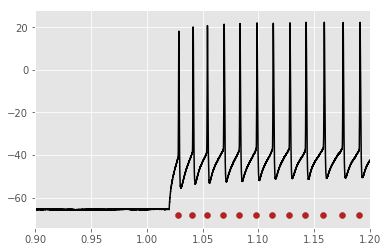We could also get the threshold voltages from the extractor and put dots where the extractor thinks the spikes begin (zooming in a little more to see better):

In :
fig = plt.figure()
plt.plot(t, v, color='black')

threshold_v = sweep_ext.spike_feature("threshold_v")

# setting zorder puts the dots on top of the trace
plt.scatter(spike_times, threshold_v, s=50, c='firebrick', zorder=20)
plt.xlim(1.015, 1.08)

Out:
(1.015, 1.08)